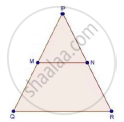Share

# M and N Are Points on the Sides Pq and Pr Respectively of a δPqr. for the Following Case, State Whether Mn || Qr Pm = 4cm, Qm = 4.5 Cm, Pn = 4 Cm and Nr = 4.5 Cm - CBSE Class 10 - Mathematics

ConceptBasic Proportionality Theorem Or Thales Theorem

#### Question

M and N are points on the sides PQ and PR respectively of a ΔPQR. For the following case, state whether MN || QR

PM = 4cm, QM = 4.5 cm, PN = 4 cm and NR = 4.5 cm

#### SolutionWe have, PM = 4cm, QM = 4.5 cm, PN = 4 cm and NR = 4.5 cm

Hence,

"PM"/"QM"=4/4.5=8/9

Also,

"PN"/"NR"=4/4.5=8/9

Hence, "PM"/"QM"="PN"/"NR"

By converse of proportionality theorem

MN || QR

Is there an error in this question or solution?

#### Video TutorialsVIEW ALL 

Solution M and N Are Points on the Sides Pq and Pr Respectively of a δPqr. for the Following Case, State Whether Mn || Qr Pm = 4cm, Qm = 4.5 Cm, Pn = 4 Cm and Nr = 4.5 Cm Concept: Basic Proportionality Theorem Or Thales Theorem.
S